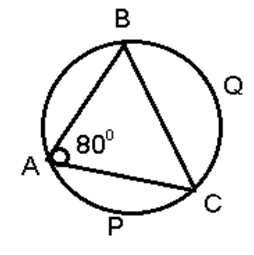SSC BOARD PAPERS IMPORTANT TOPICS COVERED FOR BOARD EXAM 2024

### GEOMETRY PAPER ONE WITH ANSWER

Q1. Attempt any 5 of the following. [5 Marks]

i. If cos θ = 1/2  find secθ . [Ans]

ii. Draw perpendicular bisector of seg AB of length 7 cm. [Ans.]

iii. If area of the circle = 105 sq. cm. and area of the minor segment = 30.75 sq. cm. Find the area of the major segment.  [Ans.]

iv. If line PQ || line AB and slope of line PQ is 3  then find the slope of line AB. [Ans]

v. ∆ DEF ~ ∆ ABC. If AB = 4 cm, DE = 3 cm, find the value of  [(∆DEF:∆ABC)] = ? [Ans]

vi. In ∆ DEF, E = 900 , If DE = 4 cm, EF = 3 cm, What is the length of side DF? [Ans]

Q2.  Attempt any four of the following. [8 marks]

i. In the adjoining figure, pint Q is on the side MP such that MQ = 2 and
MP = 5.5. Ray NQ is the bisector of MNP of ∆ MNP. Find MN:NP. [Ans]

ii. Construct ∆ PQR such that PQ = 5.7 cm, P = Q = 500. Draw RM ┴ PQ. [Ans]

iii. If cosec θ = 25/7 ,  find cot θ where θ is an acute angle. [Ans]

iv. The volume of a cue is 1000 cm3. Find its total surface area. [Ans.]

v. Find  length of the arc of the circle of radius 4.2 cm with area of the sector 18.48 sq. cm. [Ans.]

vi. In figure, if m(arcAPC) = 600, BAC = 800, find (a) ABC (b) m(arcBQC) [Ans]Q3. Attempt any three of the following [9 Marks]

i.. If 3 tan 2 θ – 43tan θ + 3 =0 then find the acute angle θ. [Ans]

ii. Construct a right angled triangle PQR where PQ = 6cm, QPR = 400, PRQ = 900 Draw circumcircle of ∆PQR. [Ans]

iii. In the figure, if LK = 63 , MK = 12, find the remaining sides of LMNK and also perimeter of LMNK. [Ans]iv. Given: A secant through point P intersects the circle in points A and B.
Tangent drawn through P touches the circle at point T. Prove that PA × PB = PT2  [Ans]

v. Find the slope of the line and also write the equation of the line passing
through the points A(- 3 , 4) and B (4 , 5). [Ans]

Q4. Solve any two: 

i. A( 3 , 7 ) , B ( 5 , 11 ), C ( -2 ,  8) are the vertices of ∆ ABC. AD is one of the median of the triangle. Find the equation of the median. [Ans]

iiThe angle of elevation of an aeroplane from a point A on the ground is 60 degree. After a flight of 15 seconds horizontally, the angle of elevation changes to 30 degree. If the aeroplane is flying at a speed of 200 m/s, then find the constant height at which the aeroplane is flying. (3 = 1.73) [Ans.]

iii. D is the midpoint of the side BC of ∆ ABC. If P and Q are points on AB and on AC such that DP bisects ∠BDA and DQ bisects ∠ADC, then prove that PQ || BC.   [Ans.]

Q5. Any Two of the folloiwing. (10 Marks)

i. In triangle PQR, given that S is a point on PQ such that ST is parallel to QR and PA:SQ = 3:5, If PR = 5.6 cm, then find PT.  [Ans. ]

ii. A vertical tree is broken by the wind. The top of the tree touches the ground and makes an angle 30 degree with it. If the top of the tree touches the ground 30 m away from its foot, then find the actual height of the tree. [Ans.]

iii. Prove the identity [cosec (90 - θ ) – sin(90 - θ ) [cosec θ – sinθ ][tanθ + cotθ ] = 1. [Ans.]